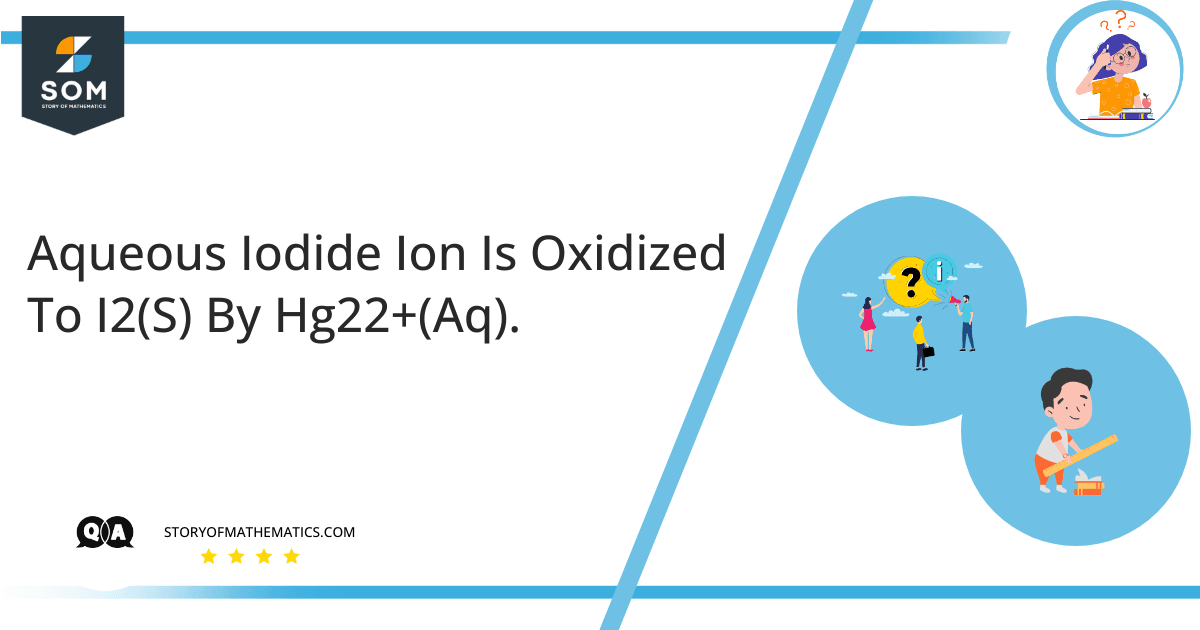# Aqueous Iodide ion is oxidized to i2(s) by hg22+(aq).This question aims to find the balanced equation and standard emf with the value of G and equilibrium constant K of the given reactions.

The quotient of the concentration of products and the concentration of reactants is expressed by equilibrium constant K while $\Delta G°$ represents the free energy during the reaction. $\Delta G°$ and K are related by the equation:

$\Delta G° = -RT lnk$

Where $\Delta G°$ shows the standard state of all reactants and products.

To find the balanced equation, we have to write the half-cell reactions:

$2I^{-1} (aq) \longrightarrow I _ 2 (s) + 2e^ {-2}$

$Hg^ {2+} _ {2} (aq) + 2e {-1} \longrightarrow 2 Hg (l)$

To write a balanced equation:

$2 I ^ {-1} (aq) + Hg^ {2+} _ {2} (aq) \longrightarrow I _ 2 (s) + 2 Hg (l)$

The term standard cell potential refers to the difference between the standard reduction potential of the cathode reaction $E ° _ {red} (cathode)$ and the standard reduction potential of the anode $E ° _ {red} (anode)$.

To find the standard cell potential:

$E °_ {cell} = E °_ {red} (cathode) – anode E °_ {red} (anode)$

$E °_ {cell} = 0.789 V – 0.536$

$E °_ {cell} = 0.253 V$

To determine the Gibbs free energy of the reaction:

$\Delta G° = – nFE°$

The symbol n represents the moles of the electrons that are transferred during the reaction while F represents Faraday’s constant.

By putting values:

$\Delta G° = – 2 mol \times 96,485( J/mol) V \times (0.253 V)$

$\Delta G° = – 48.83 kJ$

To determine the equilibrium constant, we will use the equation:

$\Delta G° = -RT lnk$

Rearranging the equation:

$lnK = \frac { – \Delta G °} { RT}$

$lnK = \frac { – 48830} { 8.314 (J/mol) K \times 298 K}$

$lnK = 19.71$

$K= e^19.71$

$K= 3.6 \times 10^8$

## Numerical Results

The answer of the balanced equation is $2 I ^ {-1} (aq) + Hg^ {2+} _ {2} (aq) \longrightarrow I _ 2 (s) + 2 Hg (l)$ and standard emf is $0.253V$ with value of G that is $-48.83 kJ$ and equilibrium constant K $3.6 \times 10^8$ of the given reactions.

## Example

To find the equilibrium constant K for the reaction of $O_2$ with $N_2$ to give NO at 423K.

The balanced equation is:

$N _ 2 ( g ) + O _ 2 ( g ) \rightleftharpoons 2 N O (g)$

$\Delta G °$ for this reaction is + 22.7 kJ /mol for $N_2$.

To determine the equilibrium constant, we will use the equation:

$\Delta G° = -RT lnk$

Rearranging the equation:

$lnK = \frac { – \Delta G °} { RT}$

$lnK = \frac { (- 22 . 7 kJ) ( 1000 J / kJ )} { 8.314 (J/mol) K \times 298 K}$

$lnK = – 6. 45$

$K= e^ – 6 . 45$

$K= 1.6 \times 10^{-3}$

Image/Mathematical drawings are created in Geogebra.